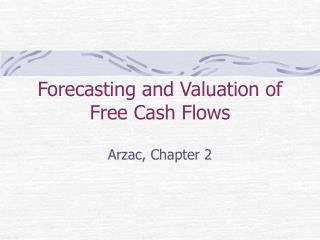Download PresentationForecasting and Valuation of Free Cash Flows

# Forecasting and Valuation of Free Cash Flows

Download Presentation## Forecasting and Valuation of Free Cash Flows

- - - - - - - - - - - - - - - - - - - - - - - - - - - E N D - - - - - - - - - - - - - - - - - - - - - - - - - - -
##### Presentation Transcript

1. Forecasting and Valuation of Free Cash Flows Arzac, Chapter 2

2. Firm Valuation • historical financial statements • forecast period • opportunity costs of capital • market value weight • make assumptions for continuation value • use formula to get value • check different scenarios

3. Firm Valuation • DCF valuation - incorporates estimates of FCF for set number of years with calculation of continuation value at end • multiples approach – comparable companies or comparable transactions

4. FCF Calculation • start with NI • add net interest expense after tax to get unlevered NI • (1-T)(Int. Exp. – Int. Inc.) = Unlevered NI • add back changes in deferred taxes and depreciation • noncash • ↑ def taxes is source of cash • depreciation can include all noncash charges deducted from EBIT except for goodwill

5. FCF Valuation • deferred taxes + unlevered NI = NOPAT • depreciation to NOPAT = Gross CF • total CF given off by firm • Gross CF – Gross Inv. = FCF (operations) • Gross Investment = • increase in WC • + capital expenditures • + investment in goodwill • + increase in net other assets

6. Financial Flows • includes all interest-earning or interest-paying financial securities and equity • independent estimate from FCF • must be equal to FCF (good check!)

7. Estimating FCF • forecast financial statements • consistency • compare with analysts ? • common forecasting error • “plugs” for building balance sheet • calculate FCF for set number of years • how long?

8. Estimating FCF • Continuation Value • idea is that over time most firms regress to industry norm • estimate FCFs over period of “competitive advantage” relative to industry and then make growth assumptions with firm converging to industry norm – i.e., constant growth

9. Estimating FCF • assumption that competition drives return on invested capital in LR to equal WACC • perpetuity model • growth rate in CF not relevant because no value creation • discount VN back to time 0 (discount using?)

10. Estimating FCF • value driver model • dominant firm in industry – Microsoft, Coca-Cola • potential to earn high returns on invested capital for very long time • discount to get value at T=0